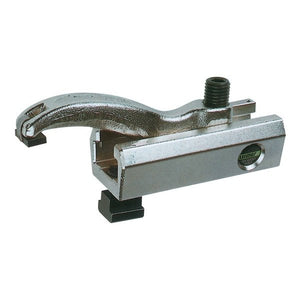## Categories# Lenzke Clamp MQ70 (to 50kN)

‚Ä¢ Patented Stepless Adjustment
‚Ä¢ Strong Clamping pressure for small clamping torque
‚Ä¢ Flexible and adjustable at every angle to the T slot
‚Ä¢ Reduction of set-up time through reliable, fast and safe clamping
‚Ä¢ Vertically highly adjustable through triple clamping shoe
‚Ä¢ Widely adjustable clamping heights through stackable clamping shoe

Thread Slot a H Comments Part no Price £ Quantity
M12 14 5-52mm V=65, L=95, L1=12, L2=-, B=52, b=24, h=37, kN=20 070-052-1214 158.84 - +
M12 14 7-72mm V=95, L=135, L1=12, L2=24, B=52, b=24, h=37, kN=22 070-072-1214 999.00 - +
M16 18 6-62mm V=75, L=110, L1=15, L2=-, B=60, b=28, h=42, kN=30 070-062-1618 999.00 - +
M16 18 8-82mm V=108, L=155, L1=15, L2=28, B=60, b=28, h=42, kN=32 070-082-1618 219.45 - +
M20 22 7-75mm V=90, L=125, L1=19, L2=-, B=75, b=34, h=48, kN=40 070-075-2022 999.00 - +
M20 22 7-92mm V=122, L=175, L1=19, L2=34, B=75, b=34, h=48, kN=42 070-092-2022 999.00 - +
M24 28 8-90mm V=112, L=150, L1=22, L2=-, B=88, b=42, h=57, kN=50 070-090-2428 999.00 - +
M24 28 8-108mm V=145, L=205, L1=22, L2=38, B=88, b=42, h=57, kN=50 070-108-2428 999.00 - +
M30 36 8-90mm V=115, L=150, L1=25, L2=-, B=88, b=42, h=57, kN=50 070-090-3036 999.00 - +
M30 36 8-108mm V=148, L=205, L1=25, L2=-, B=88, b=42, h=57, kN=50 070-108-3036 999.00 - +